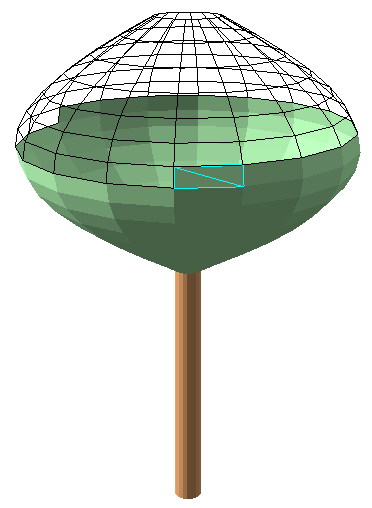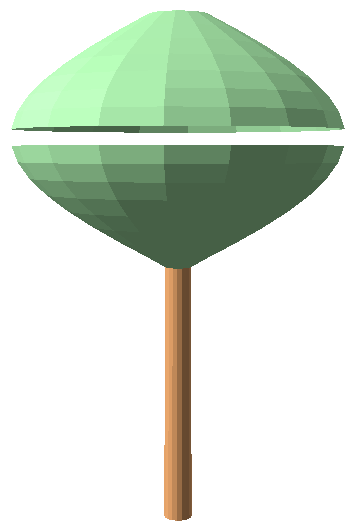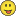# Create a 3D tree based on 3D polygons with Arcpy

290
0
07-10-2016 04:59 PM
byEsri Esteemed Contributor
1 0 290

At this point I suppose you already read my posts on Working with 3D and M-aware geometries in Arcpy​ and Creating a 3D tree with Arcpy for 3D Analysis. The idea is to create a 3D tree using polygons. Something like the one below (which is only partially shown):My first instinct was to take the code used to create the multipart polylines and divide these in parts and connect the parts from two sequential rings into a polygon. The first method I tried was to take the buffer outline and use the Polyline::positionAlongLine (value, {use_percentage}) method to pick the points on the lines. When using the same value these point should align on each ring. At least, that was what I thought. However, that was no success, leading to something very artistic, but little useful.

A very easy way of doing something similar is using the PointGeometry::pointFromAngleAndDistance (angle, distance, {method}). This yields a point based on an input point, an angle and a distance. Sweet!

The second challenge was changing the initial though of creating a multipart geometry, one for the trunk and one for the crown. This is no problem when you create a multipart polyline, but when you create a multipart polygon, it will apply some 2D topology rules to create the multipart polygon. This results in the loss of many polygon parts:The third aspect you should bear in mind is that vertical polygons will not work in Arcpy. Hence the idea: . This can be easily avoided by letting the trunk decrease in diameter as it extends upwards and by avoiding an even number of crown_circles.

When you use an even number, you get this:... a tree with a some kind of advanced ventilation implemented.So let's see what we can do to create the tree using polygons (not multipart) and throw in some python code:

`import arcpyimport mathdef main():    import os    # output    fc_out = r'C:\GeoNet\_WorkingWith3D\data.gdb\TreePolygon3D_v01'    arcpy.env.overwriteOutput = True    # the tree point    sr = arcpy.SpatialReference(3857)    x = -13046027.327    y = 4036381.962    elevation = 365    # dimensions of tree    tree_height = 30    crown_diameter = 16    trunk_diameter = 0.7    trunk_height = 15    crown_height = tree_height - trunk_height    # configuration of number of points and lines (detail of tree)    crown_lines = 15  # number of lines for crown (avoid even numbers!)    trunk_lines = 25 # number of lines for trunk    pnts_on_circle = 18  # a point every 20 degrees on circle    # create output featureclass    ws, fc_name = os.path.split(fc_out)    arcpy.CreateFeatureclass_management(ws, fc_name, "POLYGON", None, None, "ENABLED", sr)    # Addional field for tree part to store Trunk or Crown    fld_tree_part = "TreePart"    arcpy.AddField_management(fc_out, fld_tree_part, "TEXT", None, None, 10)    # insert cursor to write feature    flds_out = ('SHAPE@', fld_tree_part)    with arcpy.da.InsertCursor(fc_out, flds_out) as curs_out:        # list to store multipart feature        lst_feats = []        pnt = arcpy.Point(x, y, elevation)        pntg = arcpy.PointGeometry(pnt, sr, True)        # define z values        z0 = elevation        z1 = z0 + trunk_height        z2 = z1 + crown_height        # create list of lists with points to create trunk polygons        lst_polygons = createTrunk3Dv02(pntg, trunk_lines, pnts_on_circle, z0, z1 +1, trunk_diameter, sr)        for lst_pol in lst_polygons:            pol_trunk = arcpy.Polygon(arcpy.Array(lst_pol), sr, True, False)            row_out = (pol_trunk, "Trunk", )            curs_out.insertRow(row_out)        # create list of lists with points to create crown polygons        lst_polygons = createCrown3Dv02(pntg, crown_lines, pnts_on_circle, z1, z2, crown_diameter, sr)        for lst_pol in lst_polygons:            pol_trunk = arcpy.Polygon(arcpy.Array(lst_pol), sr, True, False)            row_out = (pol_trunk, "Crown", )            curs_out.insertRow(row_out)def createTrunk3Dv02(pntg, trunk_lines, pnts_on_circle, z0, z1, trunk_diameter, sr):    '''Create a list of lists where each inner list contains the 5 points       to create a 3D polygon as part of the trunk'''    lst_polygons = []    for a in range(pnts_on_circle):        # convert point on circle to angle 0 - 360 degrees        angle1 = float(a) / float(pnts_on_circle) * 360        angle2 = float(a + 1) / float(pnts_on_circle) * 360        # create 2 points using first and second angle and trunk diameter        pntga1d1 = pntg.pointFromAngleAndDistance(angle1, trunk_diameter, 'PLANAR')        pntga2d1 = pntg.pointFromAngleAndDistance(angle2, trunk_diameter, 'PLANAR')        # create 2 points using first and second angle and 90% of trunk diameter        pntga1d2 = pntg.pointFromAngleAndDistance(angle1, trunk_diameter * 0.9, 'PLANAR')        pntga2d2 = pntg.pointFromAngleAndDistance(angle2, trunk_diameter * 0.9, 'PLANAR')        # make points z-aware        pnta1d1 = arcpy.Point(pntga1d1.firstPoint.X, pntga1d1.firstPoint.Y, z0)        pnta2d1 = arcpy.Point(pntga2d1.firstPoint.X, pntga2d1.firstPoint.Y, z0)        pnta1d2 = arcpy.Point(pntga1d2.firstPoint.X, pntga1d2.firstPoint.Y, z1)        pnta2d2 = arcpy.Point(pntga2d2.firstPoint.X, pntga2d2.firstPoint.Y, z1)        # add 4 points + 1st point as list to polygon list        lst_polygons.append([pnta1d1, pnta2d1, pnta2d2, pnta1d2, pnta1d1])    # return list with lists of points to create polygons    return lst_polygonsdef createCrown3Dv02(pntg, crown_lines, pnts_on_circle, z1, z2, crown_diameter, sr):    '''Create a list of lists where each inner list contains the 5 points       to create a 3D polygon as part of the crown'''    lst_polygons = []    for n in range(crown_lines):        # take pairs of rings (n and n+1)        i1 = n        i2 = n + 1        # determine the elevation for each of the two rings        zi1 = float(i1) / float(crown_lines) * (z2  - z1) + z1        zi2 = float(i2) / float(crown_lines) * (z2  - z1) + z1        # convert i1 and i2 to a value expressed in pi        p1 = float(i1) / (crown_lines + 1) * math.pi        p2 = float(i2) / (crown_lines + 1) * math.pi        # calculate the diameter based on this value expressed in pi        diam1 = math.sin(p1) * float(crown_diameter)        diam2 = math.sin(p2) * float(crown_diameter)        for a in range(pnts_on_circle):            # determine the start and end angle for each pair of points on circle            angle1 = float(a) / float(pnts_on_circle) * 360            angle2 = float(a + 1) / float(pnts_on_circle) * 360            # create 4 points, two on each ring            pntga1d1 = pntg.pointFromAngleAndDistance(angle1, diam1 / 2.0, 'PLANAR')            pntga2d1 = pntg.pointFromAngleAndDistance(angle2, diam1 / 2.0, 'PLANAR')            pntga1d2 = pntg.pointFromAngleAndDistance(angle1, diam2 / 2.0, 'PLANAR')            pntga2d2 = pntg.pointFromAngleAndDistance(angle2, diam2 / 2.0, 'PLANAR')            # make points z-aware            pnta1d1 = arcpy.Point(pntga1d1.firstPoint.X, pntga1d1.firstPoint.Y, zi1)            pnta2d1 = arcpy.Point(pntga2d1.firstPoint.X, pntga2d1.firstPoint.Y, zi1)            pnta1d2 = arcpy.Point(pntga1d2.firstPoint.X, pntga1d2.firstPoint.Y, zi2)            pnta2d2 = arcpy.Point(pntga2d2.firstPoint.X, pntga2d2.firstPoint.Y, zi2)            # add 4 points + 1st point as list to polygon list            lst_polygons.append([pnta1d1, pnta2d1, pnta2d2, pnta1d2, pnta1d1])    # return list with lists of points to create polygons    return lst_polygonsif __name__ == '__main__':    main()`

If you have any questions about the code, just add a comment and I will get back to you.

Also have a look at the 3D City Base Layers - Add Trees topic of the 3D Cities section, there is a tool to create trees:

Tags (5)